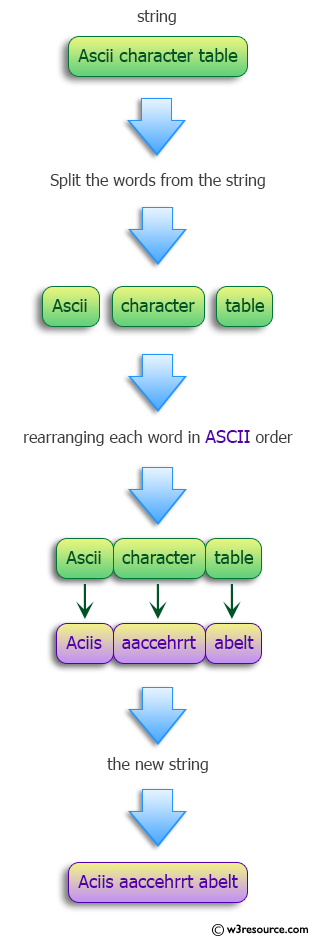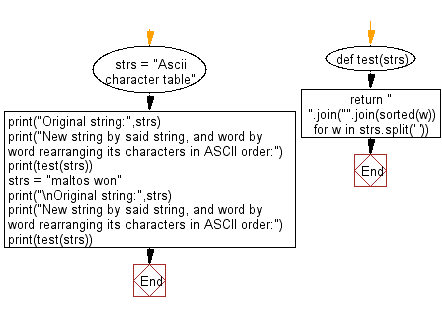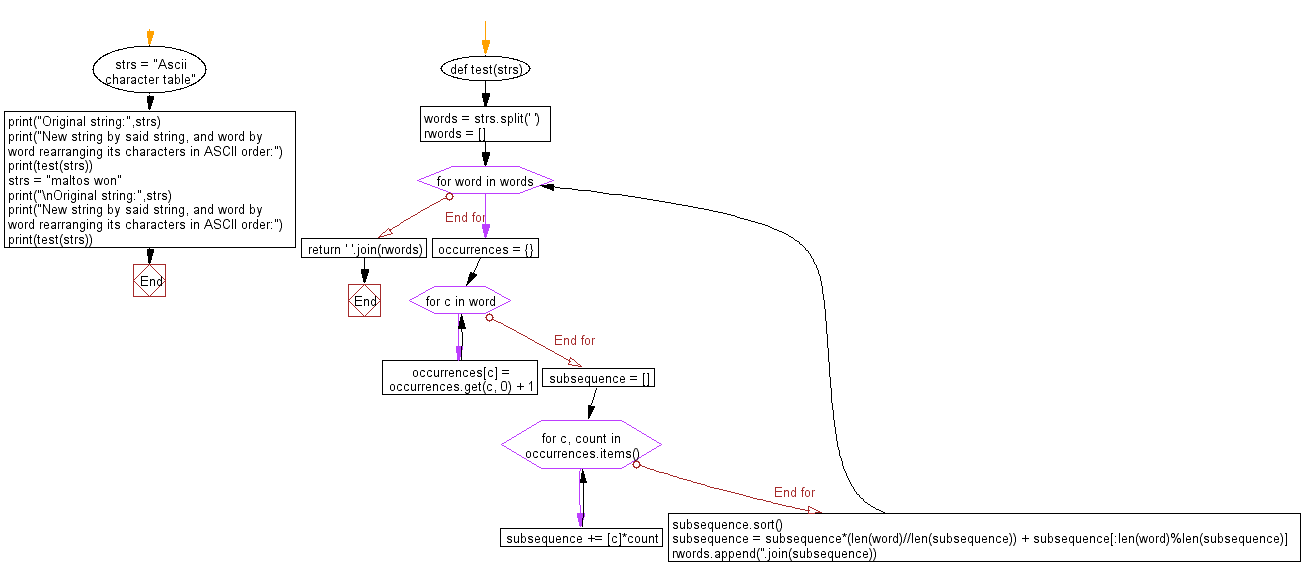﻿ Python: Create a new string by taking a string, and word by word rearranging its characters in ASCII order - w3resource# Python: Create a new string by taking a string, and word by word rearranging its characters in ASCII order

## Python Programming Puzzles: Exercise-69 with Solution

Write a Python program to create a new string by taking a string, and word by word rearranging its characters in ASCII order.

```Input: Ascii character table
Output:
Aciis aaccehrrt abelt

Input: maltos won
Output:
almost now
```

Pictorial Presentation:Sample Solution-1:

Python Code:

``````#License: https://bit.ly/3oLErEI

def test(strs):
return " ".join("".join(sorted(w)) for w in strs.split(' '))

strs = "Ascii character table"
print("Original string:",strs)
print("New string by said string, and word by word rearranging its characters in ASCII order:")
print(test(strs))
strs = "maltos won"
print("\nOriginal string:",strs)
print("New string by said string, and word by word rearranging its characters in ASCII order:")
print(test(strs))
``````

Sample Output:

```Original string: Ascii character table
New string by said string, and word by word rearranging its characters in ASCII order:
Aciis aaccehrrt abelt

Original string: maltos won
New string by said string, and word by word rearranging its characters in ASCII order:
almost now
```

Flowchart:## Visualize Python code execution:

The following tool visualize what the computer is doing step-by-step as it executes the said program:

Sample Solution-2:

Python Code:

``````#License: https://bit.ly/3oLErEI

def test(strs):
words = strs.split(' ')
rwords = []
for word in words:
occurrences = {}
for c in word:
occurrences[c] = occurrences.get(c, 0) + 1
subsequence = []
for c, count in occurrences.items():
subsequence += [c]*count
subsequence.sort()
subsequence = subsequence*(len(word)//len(subsequence)) + subsequence[:len(word)%len(subsequence)]
rwords.append(''.join(subsequence))
return ' '.join(rwords)

strs = "Ascii character table"
print("Original string:",strs)
print("New string by said string, and word by word rearranging its characters in ASCII order:")
print(test(strs))
strs = "maltos won"
print("\nOriginal string:",strs)
print("New string by said string, and word by word rearranging its characters in ASCII order:")
print(test(strs))
``````

Sample Output:

```Original string: Ascii character table
New string by said string, and word by word rearranging its characters in ASCII order:
Aciis aaccehrrt abelt

Original string: maltos won
New string by said string, and word by word rearranging its characters in ASCII order:
almost now
```

Flowchart:## Visualize Python code execution:

The following tool visualize what the computer is doing step-by-step as it executes the said program:

Python Code Editor :

Have another way to solve this solution? Contribute your code (and comments) through Disqus.

What is the difficulty level of this exercise?

Test your Programming skills with w3resource's quiz.

﻿

## Python: Tips of the Day

Clamps num within the inclusive range specified by the boundary values x and y:

Example:

```def tips_clamp_num(num,x,y):
return max(min(num, max(x, y)), min(x, y))
print(tips_clamp_num(2, 4, 6))
print(tips_clamp_num(1, -1, -6))
```

Output:

```4
-1
```